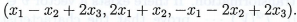【站僕】摩檸Morning>試卷(2016/09/07)

# 100 年 - 100 淡江大學 轉學考 線性代數#55896

【非選題】
1.
1. (15 points) Let T be a linear transformation from R3 to R3 defined by T【題組】 (a) Find the matrix representation of T with respect to the standard basis {(1,0,0), (0,1,0), (0,0,1)} of【非選題】
2.【題組】(b) If (a, b，c) is a vector in，what are the conditions on a, b, c that the vector is in the range of T? What is the rank of T?

【非選題】
3.【題組】(c) What are the conditions on a, b, c that the vector (a, b, c) is in the null space of T? What is the nullity of T?

【非選題】
4.2. (10 points) Let u = (2,1,0)，v = (3,0,2) and w = (0, -2,3). Suppose that T is a linear operator onthat interchanges u and v, and maps w to (1,0,0). Find the matrix representation [T]B of T with respect to the standard basis B = {(1，0,0)，(0，1，0)，(0，0，1)}.

【非選題】
5.
3.【題組】 (a) Show that for anyis linearly dependent.

【非選題】
6.【題組】(b) Show that A is invertible if and only if I belongs to Span【非選題】
7.
4. (15 points) Let T be a linear operator on a finite dimensional vector space V. Suppose T is idempotent，that is T2 = T. Prove that

【題組】 (a) Eigenvalues of T are either 0 or 1.

【非選題】
8.【題組】(b) V = ker(T)range(T).

【非選題】
9.【題組】(c) T is diagonalizable.

【非選題】
10.
3.【題組】(a) Let T : V →W be a linear transformation from vector space V to vector space W. Show that T is nonsingular (1-1) if and only if T maps a linearly independent set of vectors in F to a linearly independent set of vectors in W.

【非選題】
11.【題組】(b) Let T : V→W be a, linear transformation from vector space V to vector space W. Suppose dimV = dimVK. Show that T is one to one if and only if T is onto.

【非選題】
12.6. (15 points) Let A =. Determine whether A similar to a diagonal   matrix overIf so, exhibit a basis forsuch that A is similar to a diagonal matrix.

【非選題】
13.7. (10 points) Let A be a, n x n matrix over the fieldbe two distinct eigenvalues of A and W1,W2 be the corresponding eigenspaces forrespectively. Show that【非選題】
14.8. (10 points) Let V be a, finite dimensional vector space over a field F and dim V≥ 2. Let T : V→ 1/ be a linear transformation. If there exists a vector v G V such that V is spanned byprove that the characteristic polynomial of T is equal to it minimal polynomial.

### 懸賞詳解

#### 國二英文上第二次

22. A: Hi, George. I didn’t see you yesterday. _____________ B: I had a headache and just stayed at home the whole day. (A) Whose robot is this? (B) What was wrong? (C) Don’t go to bed late to...